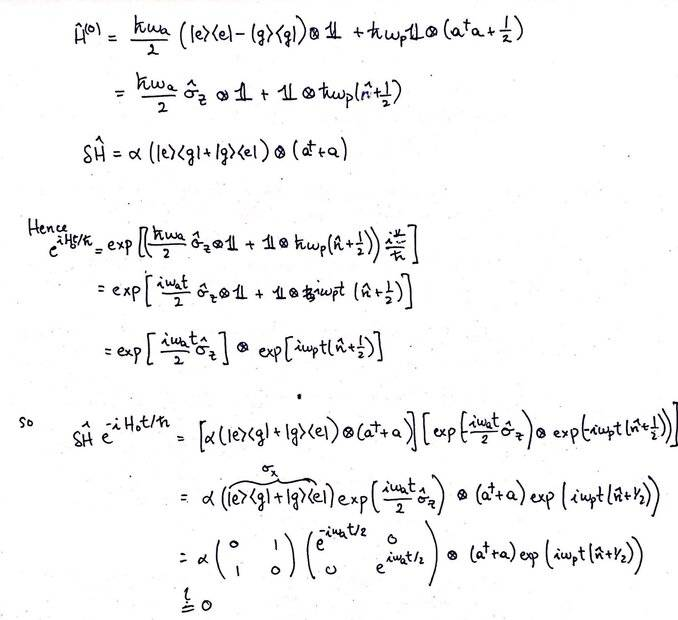# Atom-photon interactions in the interaction picture (self answered)

• I
KDPhysics
TL;DR Summary
How do we evaluate the exponential of a hamiltonian involving tensor products? Why do i get a zero result?
EDIT: I'M SO DUMB! I can't believe I can't multiply matrices together. Of course the result is not zero, the matrix on the left will be:
$$\begin{pmatrix} 0 & e^{i\omega_at/2}\\ e^{-i\omega_at/2}&0 \end{pmatrix}$$

So i was solving problem 3 from https://ocw.mit.edu/courses/physics...iii-spring-2018/assignments/MIT8_06S18ps5.pdf

Here is my working (i used properties 3 and 8 from https://en.wikipedia.org/wiki/Kronecker_product to simplify the exponential of a tensor product):Clearly 0 does not make sense, but I don't understand where I could have made a mistake? It seems like from the way the perturbation is defined as having off-diagonal elements, there is no way multiplying it by the exponential of the unperturbed hamiltonian, which is diagonal, will yield a non-zero result.

Last edited:
•Kolmo, vanhees71 and Twigg

Homework Helper
2022 Award
Also how does ##\sigma_z## get pulled through the exponential?

KDPhysics
What do you mean by pulled through?

KDPhysics
The ##\sigma_z## is a diagonal matrix so to take its exponential we can simply take the exponential of the diagonal elements.

•vanhees71 and hutchphd
Gold Member
Nice job spotting that! And don't beat yourself up over making this kind of error. It happens! I've definitely posted self-answered threads beforeI think the way you're doing is the way the problem intended you to do this. If you wanted to get fancy and see a little deeper into the physics of this process, I encourage you to rewrite the atomic part of the 0th and 1st order parts of the Hamiltonian as a vector dotted with the standard pauli matrix vector, if that makes sense (##H = H_x \sigma_x + H_y \sigma_y + H_z \sigma_z##). The interaction picture result ##\delta \tilde{H}## will have a clear geometric interpretation that way.

•vanhees71 and KDPhysics
•